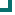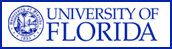Enterococcus faecalis (SOUTHEAST Data) 2004 2005 2006 2007 2008 2009 2010 penicillin 98.1%n=3380 98.3%n=2243 98.2%n=2399 98.7%n=2521 98.7%n=1327 99%n=791 98.7%n=314 ampicillin 98.8%n=2710 98.7%n=2196 78.1%n=2071 99.4%n=2721 99.3%n=1537 99.3%n=828 99.4%n=507 vancomycin 98.4%n=3717 98.3%n=2839 83.2%n=2551 98.2%n=2721 98.2%n=1536 99.5%n=826 98.6%n=507 quinupristin/dalfopristin 0.2%n=905 0.7%n=295 1.1%n=565 0.6%n=790 0%n=13 ciprofloxacin 53.3%n=458 43.1%n=295 54%n=139 52.1%n=355 51.8%n=141 58.4%n=486 49.7%n=151 levofloxacin 49.8%n=1688 46.3%n=894 56.9%n=1689 61.1%n=1647 62.4%n=595 63.5%n=586 55%n=151 nitrofurantoin 99.5%n=1277 99.2%n=1326 99.2%n=1349 99.2%n=922 98.1%n=374 99.4%n=360 96.4%n=140 tetracycline 22.7%n=784 23.8%n=1078 25.4%n=1217 19.8%n=1131 17.2%n=373 20.7%n=574 12.2%n=148 gentamicin 79.2%n=48 89.1%n=46 66.8%n=202 83.5%n=91 amikacin 99.1%n=327 97.9%n=188 imipenem 100%n=100 100%n=13 chloramphenicol 86%n=292 89.2%n=185 rifampim 86.5%n=577 81%n=295 75.4%n=122 76.5%n=451 78.3%n=120 75.3%n=691 79.3%n=309 Totals 82.3%n=16115 83.3%n=11882 73.4%n=12148 79.3%n=13461 88.4%n=6094 79.7%n=5242 83.6%n=2253

This data is accurate as of: 11/22/2016 11:47:43 AM

Drugs with less than 10 isolates are removed from the chart and from the graph. The following drugs had some data removed: gentamicin, quinupristin/dalfopristin, imipenem.ARMProgram.com is a project run by the University of Florida. Copyright 2011. All Rights Reserved. Site by JMarc Technologies, LLC